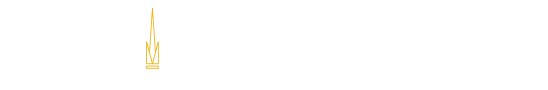### ECE Course Syllabus

### ECE3084 Course Syllabus

#### Signals and Systems (3-0-3)

Prerequisites
ECE 2025/2026 [min C] and ECE 2040 [min C]

Corequisites
None

Catalog Description
Continuous-time linear systems and signals, their mathematical representations, and computational tools; Fourier and Laplace transforms, convolutions, input-output responses, stability.

Textbook(s)
Chen, Signals and Systems (3rd edition), Oxford University Press, 2004. ISBN 0195156617, ISBN 978-0195156614 (required)

myDAQ, National Instruments. (required)

myDAQ unit, National Instruments. (required) (comment: This item is also required for ECE 2020 and ECE 2040)

Topical Outline
```1.	Introduction and motivation
a.	Engineering approximations and mathematical abstractions
b.	Continuous-time vs. discrete-time signals and systems
c.	Linear systems (superposition)
d.	Time invariance

2.	Frequency-domain signal analysis
a.	Fourier series
b.	Continuous-time Fourier transforms
c.	Properties of Fourier transforms

3.	Frequency-domain characterizations of linear systems
a.	Transfer functions (jw)
b.	Frequency responses

4.	Time-domain characterizations of linear systems
a.	Differential equations
b.	Convolution
c.	Lumped vs. distributed systems

5.	Discrete-time representations of continuous-time signals
a.	Nyquist sampling
b.	Filters (A/D -> filter -> D/A cascade)

6.	Laplace-domain signal analysis
a.	Forward and inverse Laplace transforms
b.	Properties of Laplace transforms
c.	Initial and final value theorems
d.	Convolutions
e.	Connections between Fourier and Laplace transforms

7.	Laplace-domain characterizations of linear systems
a.	States
b.	Laplace-domain representation of ODEs
c.	Transfer functions (s); poles and zeros
d.	Responses (zero state, zero input)
e.	Laplace-domain electric circuit analysis
f.	Stability
g.	Feedback

Typical in-class labs may include:
????????????  Sensing and data filtering
????????????  Proportional feedback design
????????????  Signal generation and frequency analysis

```Georgia Institute of Technology
North Avenue, Atlanta, GA 30332
Phone: 404-894-2000